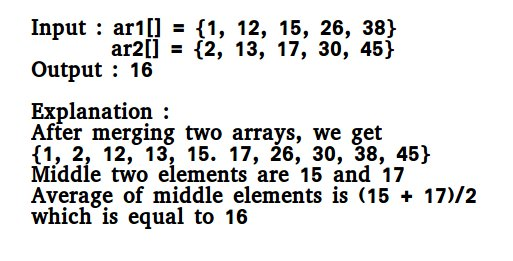Related Articles

# Python Program for Median of two sorted arrays of same size

• Last Updated : 11 Dec, 2018

There are 2 sorted arrays A and B of size n each. Write an algorithm to find the median of the array obtained merging the above 2 arrays(i.e. array of length 2n). The complexity should be O(log(n)).## Recommended: Please solve it on “PRACTICE ” first, before moving on to the solution.

Note : Since size of the set for which we are looking for median is even (2n), we need take average of middle two numbers and return floor of the average.

Method 1 (Simply count while Merging)
Use merge procedure of merge sort. Keep track of count while comparing elements of two arrays. If count becomes n(For 2n elements), we have reached the median. Take the average of the elements at indexes n-1 and n in the merged array. See the below implementation.

## Python3

 `# A Simple Merge based O(n) Python 3 solution ``# to find median of two sorted lists`` ` `# This function returns median of ar1[] and ar2[].``# Assumptions in this function:``# Both ar1[] and ar2[] are sorted arrays``# Both have n elements``def` `getMedian( ar1, ar2, n):``    ``i ``=` `0` `# Current index of i / p list ar1[]``     ` `    ``j ``=` `0` `# Current index of i / p list ar2[]``     ` `    ``m1 ``=` `-``1``    ``m2 ``=` `-``1``     ` `    ``# Since there are 2n elements, median``    ``# will be average of elements at index``    ``# n-1 and n in the array obtained after``    ``# merging ar1 and ar2``    ``count ``=` `0``    ``while` `count < n ``+` `1``:``        ``count ``+``=` `1``         ` `        ``# Below is to handle case where all``        ``# elements of ar1[] are smaller than``        ``# smallest(or first) element of ar2[]``        ``if` `i ``=``=` `n:``            ``m1 ``=` `m2``            ``m2 ``=` `ar2[``0``]``            ``break``         ` `        ``# Below is to handle case where all ``        ``# elements of ar2[] are smaller than``        ``# smallest(or first) element of ar1[]``        ``elif` `j ``=``=` `n:``            ``m1 ``=` `m2``            ``m2 ``=` `ar1[``0``]``            ``break``        ``if` `ar1[i] < ar2[j]:``            ``m1 ``=` `m2 ``# Store the prev median``            ``m2 ``=` `ar1[i]``            ``i ``+``=` `1``        ``else``:``            ``m1 ``=` `m2 ``# Store the prev median``            ``m2 ``=` `ar2[j]``            ``j ``+``=` `1``    ``return` `(m1 ``+` `m2)``/``2`` ` `# Driver code to test above function``ar1 ``=` `[``1``, ``12``, ``15``, ``26``, ``38``]``ar2 ``=` `[``2``, ``13``, ``17``, ``30``, ``45``]``n1 ``=` `len``(ar1)``n2 ``=` `len``(ar2)``if` `n1 ``=``=` `n2:``    ``print``(``"Median is "``, getMedian(ar1, ar2, n1))``else``:``    ``print``(``"Doesn't work for arrays of unequal size"``)`` ` `# This code is contributed by "Sharad_Bhardwaj".`
Output:
```Median is  16.0
```

Method 2 (By comparing the medians of two arrays)
: This method works by first getting medians of the two sorted arrays and then comparing them.

Please refer complete article on Median of two sorted arrays of same size for more details!

My Personal Notes arrow_drop_up